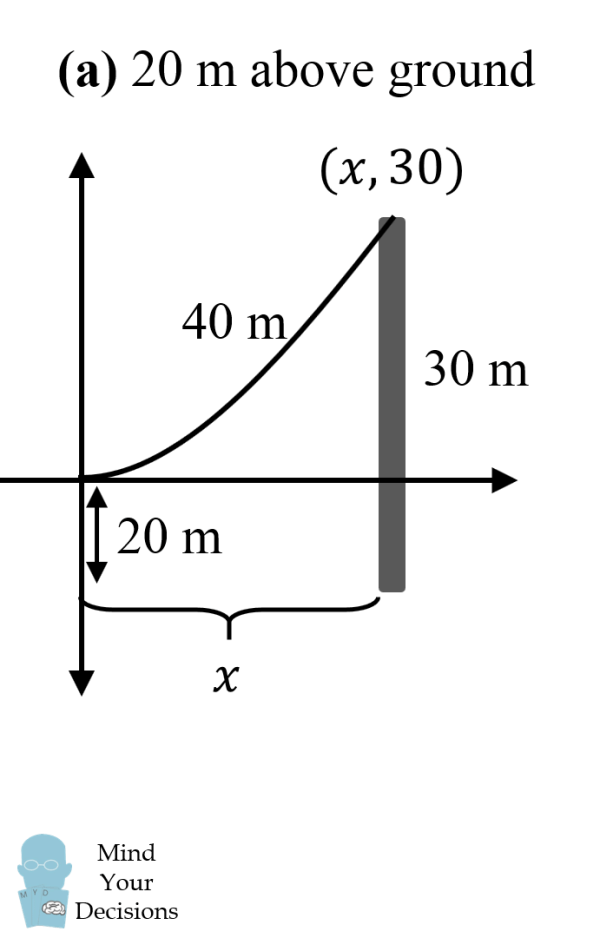August Puzzler - Digital Agent Blog
217

# August Puzzler## 24 Aug August Puzzler### Digital Agent engineers solve complex puzzles for our customers every day. Can you solve the Puzzle we created for you?

QUESTION:

A large unnamed telco company decided to install two telephone poles along the highway. Unlike Digital Agent, this company does not have front line engineers to help determine how far apart the new poles should be placed from each other. Based on the information – how far apart did they install the new telephone poles?The setup is as follows. Due to symmetry, we can consider half the problem from one pole to the center. We can then double this distance for the distance between the two poles.

Also we will use a convenient coordinate system, centered at the lowest point of the hanging cable (which takes the shape of a catenary). We get the following “half diagram”:The equation for a catenary tangent/touching the ground/x-axis is:

y = a cosh(x/a) – a
arc length = a sinh(x/a)
The parameter a is unknown, and we want to solve for the value of x of the pole.

Note the top of the pole is (x, 30), so we get one equation:
a cosh(x/a) = 30 + a

We also know that half of the cable length is 40 m, so using the equation for arc length of a catenary, we get another equation:
a sinh(x/a) = 40

We divide both equations by a to get:

cosh(x/a) = (30 + a)/a
sinh(x/a) = 40/a

We then use the hyperbolic identity:
cosh2 t – sinh2 t = 1

Substituting, we get:
(30 + a)2/a2 – (40/a)2 = 1

We can solve this to get:
a = 35/3

Then we use the equation:
(35/3) sinh (x/(35/3)) = 40
x = (35/3) arcsinh(120/35)
x = (35/3) ln(120/35 + √((120/35)2 + 1)) ≈ 22.7

As this is half the hanging chain, the distance between the two poles is then double this value:
2x = (70/3) ln(7) ≈ 45.4 m

Want to be added to our monthly Puzzler list? Fill out the information here and type “Puzzler” in the “Services of Interest” section to be added to our Puzzler email list!